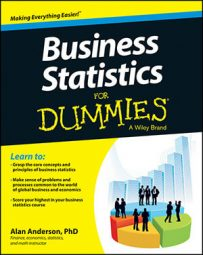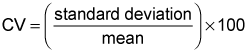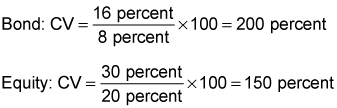Relative variation refers to the spread of a sample or a population as a proportion of the mean. Relative variation is useful because it can be expressed as a percentage, and is independent of the units in which the sample or population data are measured.

For example, you can use a measure of relative variation to compare the uncertainty or variation associated with the temperature in two different countries, even if one country uses Fahrenheit temperatures and the other uses Celsius temperatures. As another example, a measure of relative variation can be useful for comparing the returns earned by two portfolio managers. It wouldn't make any sense to compare the mean returns achieved by two different managers without explicitly considering the levels of risk that they have incurred. A measure of relative variation provides a number that considers both the risk and the return of a portfolio, so that it can be determined which portfolio is riskier relative to the return.

You can use several different types of measures of relative variation. One of the most popular is known as the coefficient of variation (CV), which indicates how "spread out" the members of a sample or population are relative to the mean. The coefficient of variation is measured as a percentage, so it's independent of the units in which the mean and standard deviation are measured. This enables the relative variation of different samples or populations to be compared directly to each other.

For example, the coefficient of variation can express the risk of an investment portfolio per unit of return. This means you can compare the performance of different portfolios to see which one offers the least amount of risk per unit of return.

Here's the formula for finding the coefficient of variation for either samples or populations:Suppose a corporation requires the services of a consulting firm to improve its accounting systems. The corporation has determined that the two best choices are Superior Accounting, Inc., and Data Services Corp. The corporation has done some research about the pricing practices of these two firms. The average price charged per hour, along with the standard deviation, are shown in the table:

Comparative Prices Charged by Superior Accounting and Data Services
Price Superior Accounting Data Services
Mean price (\$/hour) \$200 \$175
Standard deviation (\$/hour) \$80 \$75

Based on this data, the coefficient of variation for the prices charged by each firm areThese results show that although the prices charged by Superior Accounting have a larger standard deviation than Data Services, the relative variation of Data Services is greater (42.86 percent compared with 40.00 percent.) This indicates that the relative uncertainty associated with Data Services' prices is greater than for Superior Accounting's prices.

As another example, suppose a portfolio manager is responsible for an insurance company's equity portfolio and bond portfolio. He wants to know which portfolio is riskier in absolute and relative terms. He takes a sample of returns from the past ten years and computes the mean and standard deviation. This table shows the results:

Comparative Performance of Bond and Equity Portfolios
Returns Bond Portfolio Equity Portfolio
Mean return 8% 20%
Standard deviation of returns 16% 30%

These results show that the equity portfolio offers a higher average (mean) return than the bond portfolio and that the equity portfolio is riskier in absolute terms than the bond portfolio.

Because the two portfolios offer different returns and different levels of risk, it's impossible to compare them directly without using a measure of relative risk, which shows how risky a portfolio is relative to its return. So you need to find the coefficient of variation for the two portfolios, using the CV formula:The bond portfolio offers a level of risk that's 200 percent of the average return, while the equity portfolio offers a level of risk that's 150 percent of the average return. So while the equity portfolio is riskier in absolute terms (due to the higher standard deviation) the bond portfolio is riskier in relative terms (due to the higher coefficient of variation).